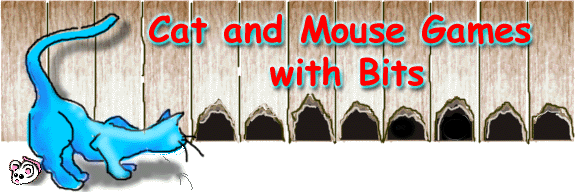Manipulation of bits is the basis behind a lot of encryption routines, data conversion between Java and other programming languages and rapid multiplication and division by 2. The barn cat has his claws into these concepts.

Every ranch has a barn, mice ... and mouse holes. Who am I? I'm the cat. They call me by some name or another, but cats only listen when they want to. I have an important job here controlling the mice and I do it bit by bit.

A computer, like the barn, has holes called bits. These holes can be occupied or not. If the hole is occupied, it has a value of 1. Otherwise, it has a value of 0. Each position in a byte represents an ever increasing value from right to left. These values double for each one. To determine what value a binary number represents, you simply add all these values together. Take this binary number for example :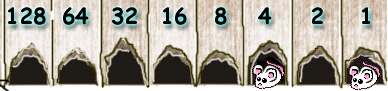4 + 1 = 5. See, it's so easy even a Cat or mouse can do it.

If you have a decimal number, there are several methods to find the binary equivalent. Perhaps the easiest is to start at the highest value of the bit. If it exceeds the value you have, it gets set to 0. Move to the next highest bit. Any bits that have a smaller or equal value are set to 1, and that value is subtracted from your number. Continue this process to the end of your byte.
Example 20.

Is 128 < or = 20 ... No, set the left most bit to 0.
Is 64 < or = 20 ... No, set to 0.
Is 32 < or = 20 ... No, set to 0.
Is 16 < or = 20 ... YES set to 1, subtract 16 from 20 leaves 4.
Is 8 < or =4 ... No, set to 0.
Is 4 < or = 4 ... Yes set to 1, subtract 4 from 4 leaves 0 so, the rest of the bits are zero.
Result =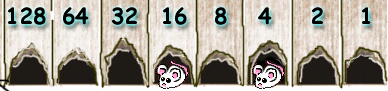Java has several operators to allow the direct manipulation of these bits. The operators are: & (And), | (Or), ^ (Xor), ~ (Inversion operator), < < (left shift), >> (signed right shift) and >>> (unsigned right shift).
Let's take a look at each operator.

First we will observe the & operator.

result = 5 & 4;

Decimal number 5 is represented by 00000101
In mouse terms this is :Decimal number 4 is represented by 00000100
In mouse terms this is :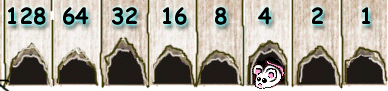Using the & operator, a bit is set in the result if and only if the bits in the same position in the operands are set.

Starting at the right, a bit is set in the first operand but not the second,
so result = 00000000.
In the second location neither is set,
so result = 00000000.
In the third position both are set, so the bit gets set,
so result = 00000100.
For the remaining positions neither is set,
so the result remains 00000100.What happens to the other mouse? Well, Ranch hands just never see it again. Yummm.

Using the same values, the | operator works like this:

result = 5 | 4 ;

A bit is set in the result if the bit is set in either operand.

Starting at the right, we see that the bit is set in the first operand, so a bit gets set in the result even though the bit isn't set in that position in the second operand,
so result = 00000001.
The bit isn't set in the second position in either byte,
so result = 00000001.
In the third position, the bit is set in both operands, so the bit gets set,
so result = 00000101.
Since none of the remaining bits are set in the remaining positions,
result remains 00000101.Don't say it. One gets away every now and then. Your point is????

Likewise demonstrating the ^ operator:

result = 5 ^ 4 ;

A bit gets set in the result if it is set in one of the operands but not the other.

The first bit is set in the top but not the bottom
so result = 00000001.
Since the second isn't set in either, it doesn't get set in the result
so result = 00000001.
The third bit is set in both, which means it doesn't get set in the result
so result = 00000001.
In the final positions, it's not set in either,
so result = 00000001.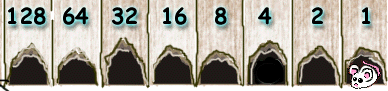Unlike the others, the ~ operator only operates on one operand to return a result. It's written as:

result = ~5 ;

Any bits in the operand that are 0 are set to 1 in the result, and any that were 1 are set to 0. So starting with this pattern:The result looks like this: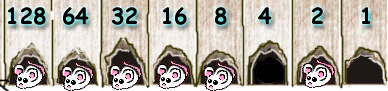A whole lot of mice makes a nice lunch. :)

This brings us to shifting. You can think of this as mice jumping from hole to hole trying to escape.

In the < < left shift all bytes are moved to the left the number of places you specify.

For example:

result = 5 << 2 ;

is visualized thus:Signed right shift ( >> ) and unsigned right shift ( >>> ) work identically for positive numbers. So looking at

result = 20 >> 2 ;
or
result = 20 >>> 2 ;

we see that the operand 20 looks like this:and result = 5 looks like this.Negative numbers, however, are treated differently.

With >>> , all bits are shifted to the right, and zeros fill in the leftmost bits.

For example:

result = -1 >>> 2 ;

The operand -1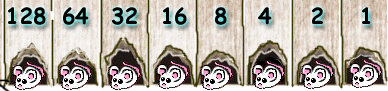becomes:But >> shifts the bits to the right using zeros to fill the leftmost bits, and when it finishes, it resets the left bit to 1 to keep the result negative.

So result = -99 >> 2 ;

looks like this:result:The mouse that moved off the right side... It is gone for all time :)

Signed right shift has some interesting side effects when shifting negative numbers.

Lets look at result = -1 >> 2 ;

operand:result:The bit arrangement didn't change! The result is still -1. This is because java does this in steps, one bit at a time. Move all bits to the right one, fill in the left bit with a 1. Move all bits to the right a second time and fill in the left bit with a 1. In fact:

result = -128 >> 7 ;

operand: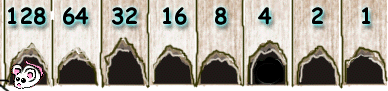result:Signed right shift will propagate the left most one through the rest of the bits!

When you shift a bit by a numeric value greater than the size in bits, Java does a modulas shift. In our examples, we've been doing shifts on one byte eight bits. So, if we were to shift by 9,

result = 5 << 9 ;

operand:Java shifts this 9 % 8 or 1.

result: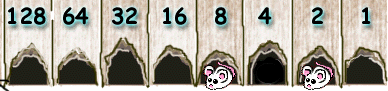I am only a Cat and can only think in small terms. So all the examples used were based on 8 bits. Java, on the other hand, does it's operations based on the int data type. In reality, the operands would first be cast to an int (32 bits). If you want the result as another type, an explicit cast is required.

For more details on this, read the Cup Size Campfire story.